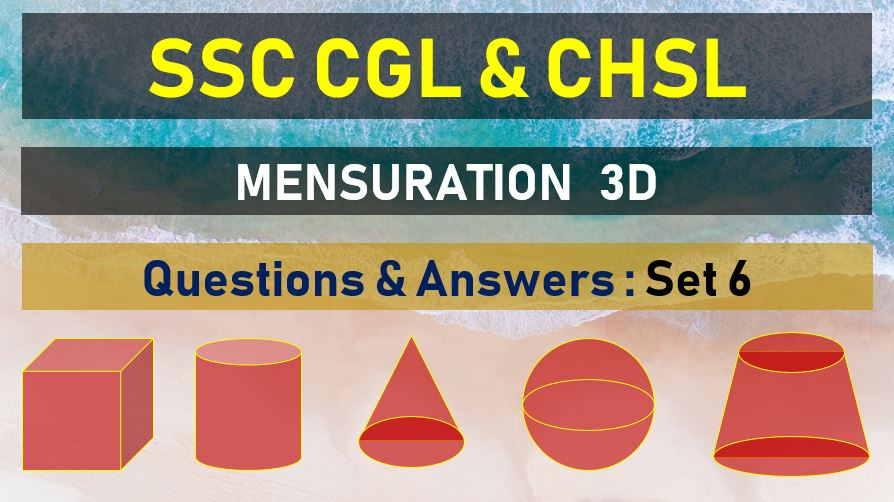Tuesday, June 22, 2021
Home > mensuration > SSC CHSL Surface Area and Volume Questions Answers Set 6

# SSC CHSL Surface Area and Volume Questions Answers Set 6SSC Mensurations 3D
Surface Area & Volume Questions Sets
Set 1Set 2Set 3Set 4Set 5
Set 6Set 7Set 8Set 9Set 10

SSC CHSL Surface Area and Volume Questions Answers Set 6: Ques No 1

If the slant height of a right pyramid with square base is 4 meter and the total slant surface of the pyramid is 12 sq. m. then the ratio of total slant surface and area of the base is

Options:

A. 16 : 3

B. 24 : 5

C. 32 : 9

D. 12 : 3

Answer: A

Solution:

SSC CHSL Surface Area and Volume Questions Answers Set 6: Ques No 2

A prism has as the base a right angled triangle whose sides adjacent to the right angles are 10 cm and 12 cm long. The height of the prism is 20 cm. the density of the material of the prism is 6 gm /cubic cm. the weight of the prism is

Options:

A. 6.4 kg

B. 7.2 kg

C. 3.4 kg

D. 4.8 kg

Answer: B

Solution:

SSC CHSL Surface Area and Volume Questions Answers Set 6: Ques No 3

The surface area of a sphere of radius 5 cm is five times the area of the curved surface of cone of radius 4 cm. Find the height of the cone.

Options:

A. 3 cm

B. 2 cm

C. 4 cm

D. 2 cm

Answer: A

Solution:

SSC CHSL Surface Area and Volume Questions Answers Set 6: Ques No 4

The base of a right prism is an equilateral triangle of side 8 cm and height of the prism is 10 cm. Then the volume of the prism is

Options:

A. 320√3 cubic cm

B. 160√3 cubic cm

C. 150√3 cubic cm

D. 300√3 cubic cm

Answer: B

Solution:

SSC CHSL Surface Area and Volume Questions Answers Set 6: Ques No 5

The height of a right prism with a square base is 15 cm, If the area of the total surface of the prism is 608 sq. cm, its volume is

Options:

A. 910 cm3

B. 920 cm3

C. 960 cm3

D. 980 cm3

Answer: C

Solution:

SSC CHSL Surface Area and Volume Questions Answer Set 6: Ques No 6

Water in a canel, 30 dm wide and 12 dm deep, is flowing with a speed of 10 km/hour. How much area will it irrigate in 30 minutes, if 8 cm of standing water is required for irrigation.

Options:

A. 220500 cm2

B. 22000 cm2

C. 22500 cm2

D. 225000 cm2

Answer: D

Solution:

SSC CHSL Surface Area and Volume Questions Answer Set 6: Ques No 7

Area of the base of a pyramid is 57 sq. cm and height is 10 cm, then its volume (in cm3), is

Options:

A. 570

B. 390

C. 190

D. 390

Answer: C

Solution:

SSC CHSL Surface Area and Volume Questions Answer Set 6: Ques No 8

The base of a right pyramid is a square of side 16 cm long. If its height be 15 cm , then the area of the lateral surface in square cm is:

Options:

A. 136

B. 544

C. 800

D. 1280

Answer: B

Solution:

SSC CHSL Surface Area and Volume Questions Answer Set 6: Ques No 9

How many spherical bullets can be made out of a solid cube of lead whose edge measures 44 cm, each bullet being 4 cm in diameter.

Options:

A. 2451

B. 2541

C. 2304

D. 2536

Answer: B

Solution:

SSC CHSL Surface Area and Volume Questions Answer Set 6: Ques No 10

The base of a right prism is an equilateral triangle of area 173 cm2 and the volume of the prism is10380 cm2. The area of the lateral surface of the prism is

Options:

A. 1200 cm2

B. 2400 cm2

C. 3600 cm2

D. 4380 cm2

Answer: C

Solution:

SSC Mensurations 3D
Surface Area & Volume Questions Sets
Set 1Set 2Set 3Set 4Set 5
Set 6Set 7Set 8Set 9Set 10
error: Content is protected !!